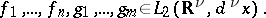# Annihilation operators

Jump to: navigation, search

A family of closed linear operators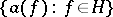, whereis some Hilbert space, acting on a Fock space constructed from(i.e. on the symmetrization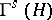or anti-symmetrization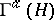of the space of tensors over) such that on the vector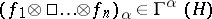,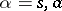, consisting of the symmetrized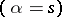or anti-symmetrized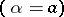tensor product of a sequence of elements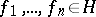,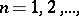in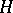, they are given by the formulas: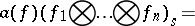(1)in the symmetric case, and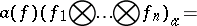(2)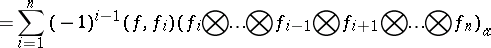in the anti-symmetric case; the empty vector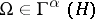,(i.e. the unit vector in the subspace of constants in) is mapped to zero by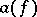. In these formulasis the inner product in. The operators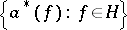dual to the operatorsare called creation operators; their action on the vectors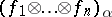,,is given by the formulas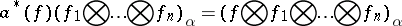(3)

andAs a consequence of these definitions, for each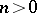the subspace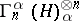,, the symmetrized or anti-symmetrized-th tensor power of, is mapped byinto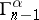and by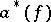into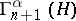.

In quantum physics, the Fock space,, is interpreted as the state space of a system consisting of an arbitrary (finite) number of identical quantum particles, the spaceis the state space of a single particle, the subspacecorresponds to the states of the system withparticles, i.e. states in which there are justparticles. A state withparticles is mapped byto a state with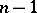particles ( "annihilation" of a particle), and byto a state withparticles ( "creation" of a particle).

The operatorsandform irreducible families of operators satisfying the following permutation relations: In the symmetric case (the commutation relations)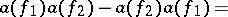(4)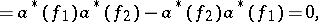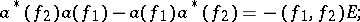and in the anti-symmetric case (the anti-commutation relations)(5)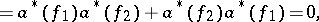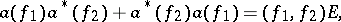where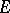is the identity operator inor. Besides the families of operatorsand,, described here, there exist in the case of an infinite-dimensional spacealso other irreducible representations of the commutation and anti-commutation relations (4) and (5), not equivalent to those given above. Sometimes they are also called creation and annihilation operators. In the case of a finite-dimensional space, all irreducible representations of the commutation or anti-commutation relations are unitarily equivalent.

The operators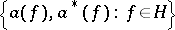are in many connections convenient "generators" in the set of all linear operators acting in the space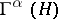,, and the representation of such operators as the sum of arbitrary creation and annihilation operators (the normal form of an operator) is very useful in applications. The connection with this formalism bears the name method of second quantization, cf. .

In the particular, but for applications important, case in which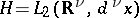,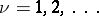(or in a more general case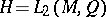, whereis a measure space), the family of operatorsdefines two operator-valued generalized functions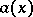andsuch thatThe introduction ofandturns out to be convenient for the formalism of second quantization (e.g. it allows one directly to consider operators of the form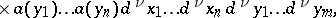where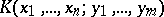is a certain "sufficiently-good" function), without having to recourse to their decomposition as a series in the monomials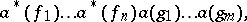where